# SI Core Engine

Spark-ignition engine from intake to exhaust port

• Library:
• Powertrain Blockset / Propulsion / Combustion Engine Components / Core Engine

•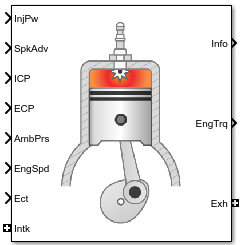## Description

The SI Core Engine block implements a spark-ignition (SI) engine from intake to exhaust port. You can use the block in larger vehicle models, hardware-in-the-loop (HIL) engine control design, or vehicle-level fuel economy and performance simulations.

The SI Core Engine block calculates:

• Brake torque

• Fuel flow

• Port gas mass flow, including exhaust gas recirculation (EGR)

• Air-fuel ratio (AFR)

• Exhaust temperature and exhaust mass flow rate

• Engine-out (EO) exhaust emissions

• Hydrocarbon (HC)

• Carbon monoxide (CO)

• Nitric oxide and nitrogen dioxide (NOx)

• Carbon dioxide (CO2)

• Particulate matter (PM)

### Air Mass Flow

To calculate engine air mass flow, configure the SI engine to use either of these air mass flow models.

Air Mass Flow ModelDescription
SI Engine Speed-Density Air Mass Flow Model

Uses the speed-density equation to calculate the engine air mass flow, relating the engine air mass flow to the intake manifold pressure and engine speed. Consider using this air mass flow model in engines with fixed valvetrain designs.

SI Engine Dual-Independent Cam Phaser Air Mass Flow Model

To calculate the engine air mass flow, the dual-independent cam phaser model uses:

• Empirical calibration parameters developed from engine mapping measurements

• Desktop calibration parameters derived from engine computer-aided design (CAD) data

In contrast to typical embedded air mass flow calculations based on direct air mass flow measurement with an air mass flow (MAF) sensor, this air mass flow model offers:

• Elimination of MAF sensors in dual cam-phased valvetrain applications

• Reasonable accuracy with changes in altitude

• Semiphysical modeling approach

• Bounded behavior

• Suitable execution time for electronic control unit (ECU) implementation

• Systematic development of a relatively small number of calibration parameters

### Brake Torque

To calculate the brake torque, configure the SI engine to use either of these torque models.

Brake Torque ModelDescription
SI Engine Torque Structure Model

For the structured brake torque calculation, the SI engine uses tables for the inner torque, friction torque, optimal spark, spark efficiency, and lambda efficiency.

If you select Crank angle pressure and torque on the block Torque tab, you can:

• Simulate advanced closed-loop engine controls in desktop simulations and on HIL bench, based on cylinder pressure recorded from a model or laboratory test as a function of crank angle.

• Simulate driveline vibrations downstream of the engine due to high-frequency crankshaft torsionals.

• Simulate engine misfires due to lean operation or spark plug fouling by using the injector pulse width input.

• Simulate cylinder deactivation effect (closed intake and exhaust valves, no injected fuel) on individual cylinder pressures, mean-value airflow, mean-value torque, and crank-angle-based torque.

• Simulate the fuel-cut effect on individual cylinder pressure, mean-value torque, and crank-angle-based torque.

SI Engine Simple Torque Model

For the simple brake torque calculation, the SI engine block uses a torque lookup table map that is a function of engine speed and load.

### Fuel Flow

To calculate the fuel flow, the SI Core Engine block uses fuel injector characteristics and fuel injector pulse-width.

`${\stackrel{˙}{m}}_{fuel}=\frac{N{S}_{inj}P{w}_{inj}{N}_{cyl}}{Cps\left(\frac{60s}{\mathrm{min}}\right)\left(\frac{1000mg}{g}\right)}$`

To calculate the fuel economy for high-fidelity models, the block uses the volumetric fuel flow.

`${Q}_{fuel}=\frac{{\stackrel{˙}{m}}_{fuel}}{\left(\frac{1000kg}{{m}^{3}}\right)S{g}_{fuel}}$`

The equation uses these variables.

 ${\stackrel{˙}{m}}_{fuel}$ Fuel mass flow, g/s $\omega$ Engine rotational speed, rad/s $Cps$ Crankshaft revolutions per power stroke, rev/stroke ${S}_{inj}$ Fuel injector slope, mg/ms $P{w}_{inj}$ Fuel injector pulse-width, ms ${N}_{cyl}$ Number of engine cylinders N Engine speed, rpm Sgfuel Specific gravity of fuel Qfuel Volumetric fuel flow

The block uses the internal signal `FlwDir` to track the direction of the flow.

### Air-Fuel Ratio

To calculate the air-fuel (AFR) ratio, the CI Core Engine and SI Core Engine blocks implement this equation.

`$AFR=\frac{{\stackrel{˙}{m}}_{air}}{{\stackrel{˙}{m}}_{fuel}}$`

The CI Core Engine uses this equation to calculate the relative AFR.

`$\lambda =\frac{AFR}{AF{R}_{s}}$`

To calculate the exhaust gas recirculation (EGR), the blocks implement this equation. The calculation expresses the EGR as a percent of the total intake port flow.

`$EG{R}_{pct}=100\frac{{\stackrel{˙}{m}}_{intk,b}}{{\stackrel{˙}{m}}_{intk}}=100{y}_{intk,b}$`

The equations use these variables.

 $AFR$ Air-fuel ratio AFRs Stoichiometric air-fuel ratio ${\stackrel{˙}{m}}_{intk}$ Engine air mass flow ${\stackrel{˙}{m}}_{fuel}$ Fuel mass flow λ Relative AFR yintk,b Intake burned mass fraction EGRpct EGR percent ${\stackrel{˙}{m}}_{intk,b}$ Recirculated burned gas mass flow rate

### Exhaust

The block calculates the:

• Exhaust gas temperature

• Exhaust gas-specific enthalpy

• Exhaust gas mass flow rate

• Engine-out (EO) exhaust emissions:

• Hydrocarbon (HC)

• Carbon monoxide (CO)

• Nitric oxide and nitrogen dioxide (NOx)

• Carbon dioxide (CO2)

• Particulate matter (PM)

The exhaust temperature determines the specific enthalpy.

`${h}_{exh}=C{p}_{exh}{T}_{exh}$`

The exhaust mass flow rate is the sum of the intake port air mass flow and the fuel mass flow.

`${\stackrel{˙}{m}}_{exh}={\stackrel{˙}{m}}_{intake}+{\stackrel{˙}{m}}_{fuel}$`

To calculate the exhaust emissions, the block multiplies the emission mass fraction by the exhaust mass flow rate. To determine the emission mass fractions, the block uses lookup tables that are functions of the engine torque and speed.

`$\begin{array}{l}{y}_{exh,i}={f}_{i_frac}\left({T}_{brake},N\right)\\ {\stackrel{˙}{m}}_{exh,i}={\stackrel{˙}{m}}_{exh}{y}_{exh,i}\end{array}$`

The fraction of air and fuel entering the intake port, injected fuel, and stoichiometric AFR determine the air mass fraction that exits the exhaust.

`${y}_{exh,air}=\mathrm{max}\left[{y}_{in,air}-\frac{{\stackrel{˙}{m}}_{fuel}+{y}_{in,fuel}{\stackrel{˙}{m}}_{intake}}{{\stackrel{˙}{m}}_{fuel}+{\stackrel{˙}{m}}_{intake}}AF{R}_{s}\right]$`

If the engine is operating at the stoichiometric or fuel rich AFR, no air exits the exhaust. Unburned hydrocarbons and burned gas comprise the remainder of the exhaust gas. This equation determines the exhaust burned gas mass fraction.

`${y}_{exh,b}=\mathrm{max}\left[\left(1-{y}_{exh,air}-{y}_{exh,HC}\right),0\right]$`

The equations use these variables.

 ${T}_{exh}$ Engine exhaust temperature ${h}_{exh}$ Exhaust manifold inlet-specific enthalpy $C{p}_{exh}$ Exhaust gas specific heat ${\stackrel{˙}{m}}_{intk}$ Intake port air mass flow rate ${\stackrel{˙}{m}}_{fuel}$ Fuel mass flow rate ${\stackrel{˙}{m}}_{exh}$ Exhaust mass flow rate ${y}_{in,fuel}$ Intake fuel mass fraction yexh,i Exhaust mass fraction for i = CO2, CO, HC, NOx, air, burned gas, and PM ${\stackrel{˙}{m}}_{exh,i}$ Exhaust mass flow rate for i = CO2, CO, HC, NOx, air, burned gas, and PM Tbrake Engine brake torque N Engine speed yexh,air Exhaust air mass fraction yexh,b Exhaust air burned mass fraction

### Power Accounting

For the power accounting, the block implements equations that depend on Torque model.

When you set Torque model to `Simple Torque Lookup`, the block implements these equations.

Bus Signal DescriptionEquations

`PwrInfo`

`PwrTrnsfrd` — Power transferred between blocks

• Positive signals indicate flow into block

• Negative signals indicate flow out of block

`PwrIntkHeatFlw`

Intake heat flow

${\stackrel{˙}{m}}_{intk}{h}_{intk}$

`PwrExhHeatFlw`

Exhaust heat flow

$-{\stackrel{˙}{m}}_{exh}{h}_{exh}$

`PwrCrkshft`Crankshaft power

$-{T}_{brake}\omega$

`PwrNotTrnsfrd` — Power crossing the block boundary, but not transferred

• Positive signals indicate an input

• Negative signals indicate a loss

`PwrFuel`

Fuel input power

${\stackrel{˙}{m}}_{fuel}LHV$

`PwrLoss`

All losses

${T}_{brake}\omega -{\stackrel{˙}{m}}_{fuel}LHV-{\stackrel{˙}{m}}_{intk}{h}_{intk}+{\stackrel{˙}{m}}_{exh}{h}_{exh}$

`PwrStored` — Stored energy rate of change

• Positive signals indicate an increase

• Negative signals indicate a decrease

Not used

When you set Torque model to `Torque Structure`, the block implements these equations.

Bus Signal DescriptionEquations

`PwrInfo`

`PwrTrnsfrd` — Power transferred between blocks

• Positive signals indicate flow into block

• Negative signals indicate flow out of block

`PwrIntkHeatFlw`

Intake heat flow

${\stackrel{˙}{m}}_{intk}{h}_{intk}$

`PwrExhHeatFlw`

Exhaust heat flow

$-{\stackrel{˙}{m}}_{exh}{h}_{exh}$

`PwrCrkshft`Crankshaft power

$-{T}_{brake}\omega$

`PwrNotTrnsfrd` — Power crossing the block boundary, but not transferred

• Positive signals indicate an input

• Negative signals indicate a loss

`PwrFuel`

Fuel input power

${\stackrel{˙}{m}}_{fuel}LHV$

`PwrFricLoss`

Friction loss

$-{T}_{fric}\omega$

`PwrPumpLoss`

Pumping loss

$-{T}_{pump}\omega$

`PwrHeatTrnsfrLoss`

Heat transfer loss

${T}_{brake}\omega -{\stackrel{˙}{m}}_{fuel}LHV-{\stackrel{˙}{m}}_{intk}{h}_{intk}+{\stackrel{˙}{m}}_{exh}{h}_{exh}+{T}_{fric}\omega +{T}_{pump}\omega$

`PwrStored` — Stored energy rate of change

• Positive signals indicate an increase

• Negative signals indicate a decrease

Not used

 hexh Exhaust manifold inlet-specific enthalpy hintk Intake port specific enthalpy ${\stackrel{˙}{m}}_{intk}$ Intake port air mass flow rate ${\stackrel{˙}{m}}_{fuel}$ Fuel mass flow rate ${\stackrel{˙}{m}}_{exh}$ Exhaust mass flow rate ω Engine speed Tbrake Brake torque Tpump Engine pumping work offset to inner torque Tfric Engine friction torque LHV Fuel lower heating value

## Ports

### Input

expand all

Fuel injector pulse-width, $P{w}_{inj}$, in ms.

Spark advance, SA, in degrees crank angle before top dead center (degBTDC).

#### Dependencies

To create this port, for the Torque model parameter, select `Torque Structure`.

Intake cam phase angle command, ${\phi }_{ICPCMD}$, in degCrkAdv, or degrees crank advance.

#### Dependencies

To create this port, for the Air mass flow model parameter, select `Dual-Independent Variable Cam Phasing`.

Exhaust cam phase angle command, ${\phi }_{ECPCMD}$, in degCrkRet, or degrees crank retard.

#### Dependencies

To create this port, for the Air mass flow model parameter, select `Dual-Independent Variable Cam Phasing`.

Ambient pressure, ${P}_{Amb}$, in Pa.

#### Dependencies

To create this port, for the Air mass flow model parameter, select `Dual-Independent Variable Cam Phasing`.

Engine speed, N, in rpm.

Engine cooling temperature, Tcoolant, in K.

#### Dependencies

To enable this parameter, for Torque model, select `Torque Structure`.

Bus containing the upstream:

• `Prs` — Pressure, in Pa

• `Temp` — Temperature, in K

• `Enth` — Specific enthalpy, in J/kg

• `MassFrac` — Intake port mass fractions, dimensionless. EGR mass flow at the intake port is burned gas.

Specifically, a bus with these mass fractions:

• `O2MassFrac` — Oxygen

• `N2MassFrac` — Nitrogen

• `UnbrndFuelMassFrac` — Unburned fuel

• `CO2MassFrac` — Carbon dioxide

• `H2OMassFrac` — Water

• `COMassFrac` — Carbon monoxide

• `NOMassFrac` — Nitric oxide

• `NO2MassFrac` — Nitrogen dioxide

• `NOxMassFrac` — Nitric oxide and nitrogen dioxide

• `PmMassFrac` — Particulate matter

• `AirMassFrac` — Air

• `BrndGasMassFrac` — Burned gas

Bus containing the exhaust:

• `Prs` — Pressure, in Pa

• `Temp` — Temperature, in K

• `Enth` — Specific enthalpy, in J/kg

• `MassFrac` — Exhaust port mass fractions, dimensionless.

Specifically, a bus with these mass fractions:

• `O2MassFrac` — Oxygen

• `N2MassFrac` — Nitrogen

• `UnbrndFuelMassFrac` — Unburned fuel

• `CO2MassFrac` — Carbon dioxide

• `H2OMassFrac` — Water

• `COMassFrac` — Carbon monoxide

• `NOMassFrac` — Nitric oxide

• `NO2MassFrac` — Nitrogen dioxide

• `NOxMassFrac` — Nitric oxide and nitrogen dioxide

• `PmMassFrac` — Particulate matter

• `AirMassFrac` — Air

• `BrndGasMassFrac` — Burned gas

### Output

expand all

Bus signal that contains these block calculations.

SignalDescriptionVariableUnits

`IntkGasMassFlw`

Engine intake air mass flow

${\stackrel{˙}{m}}_{air}$

kg/s

`IntkAirMassFlw`

Engine intake port mass flow

${\stackrel{˙}{m}}_{intk}$

kg/s

`NrmlzdAirChrg`

Engine load (that is, normalized cylinder air mass) corrected for final steady-state cam phase angles

$L$N/A

`Afr`

Air-fuel ratio at engine exhaust port

$AFR$N/A

`FuelMassFlw`

Fuel flow into engine

${\stackrel{˙}{m}}_{fuel}$kg/s

`FuelVolFlw`

Volumetric fuel flow

Qfuel

m3/s

`ExhManGasTemp`

Exhaust gas temperature at exhaust manifold inlet

${T}_{exh}$K

`EngTrq`

Engine brake torque

${T}_{brake}$N·m

`EngSpd`

Engine speed

$N$rpm

`IntkCamPhase`

Intake cam phaser angle

${\phi }_{ICP}$ i

`ExhCamPhase`

Exhaust cam phaser angle

${\phi }_{ECP}$

degrees crank retard

`CrkAng`

Engine crankshaft absolute angle

$\underset{0}{\overset{\left(360\right)Cps}{\int }}EngSpd\frac{180}{30}d\theta$

where $Cps$ is crankshaft revolutions per power stroke

degrees crank angle

`EgrPct`

EGR percent

EGRpct

N/A

`EoAir`

EO air mass flow rate

${\stackrel{˙}{m}}_{exh}$

kg/s

`EoBrndGas`

EO burned gas mass flow rate

yexh,b

kg/s

`EoHC`

EO hydrocarbon emission mass flow rate

yexh,HC

kg/s

`EoCO`

EO carbon monoxide emission mass flow rate

yexh,CO

kg/s

`EoNOx`

EO nitric oxide and nitrogen dioxide emissions mass flow rate

yexh,NOx

kg/s

`EoCO2`

EO carbon dioxide emission mass flow rate

yexh,CO2

kg/s

`EoPm`

EO particulate matter emission mass flow rate

yexh,PM

kg/s

`CylPrs`

Cylinder pressure

N/A

Pa

`EngTrqCrk`

Crank-angle based engine torque

N/A

N·m

`PwrInfo``PwrTrnsfrd`

`PwrIntkHeatFlw`

Intake heat flow

${\stackrel{˙}{m}}_{intk}{h}_{intk}$

W
`PwrExhHeatFlw`

Exhaust heat flow

$-{\stackrel{˙}{m}}_{exh}{h}_{exh}$

W
`PwrCrkshft`Crankshaft power

$-{T}_{brake}\omega$

W
`PwrNotTrnsfrd``PwrFuel`

Fuel input power

${\stackrel{˙}{m}}_{fuel}LHV$

W
`PwrLoss`

For Torque model set to ```Simple Torque Lookup```:

All losses

${T}_{brake}\omega -{\stackrel{˙}{m}}_{fuel}LHV-{\stackrel{˙}{m}}_{intk}{h}_{intk}+{\stackrel{˙}{m}}_{exh}{h}_{exh}$

W
`PwrFricLoss`

For Torque model set to ```Torque Structure```:

Friction loss

$-{T}_{fric}\omega$

W
`PwrPumpLoss`

For Torque model set to ```Torque Structure```:

Pumping loss

$-{T}_{pump}\omega$

W
`PwrHeatTrnsfrLoss`

For Torque model set to ```Torque Structure```:

Heat transfer loss

${T}_{brake}\omega -{\stackrel{˙}{m}}_{fuel}LHV-{\stackrel{˙}{m}}_{intk}{h}_{intk}+{\stackrel{˙}{m}}_{exh}{h}_{exh}+{T}_{fric}\omega +{T}_{pump}\omega$

W
`PwrStored`Not used

Engine brake torque, ${T}_{brake}$, in N·m.

Bus containing:

• `MassFlwRate` — Intake port mass flow rate, in kg/s

• `HeatFlwRate` — Intake port heat flow rate, in J/s

• `Temp` — Intake port temperature, in K

• `MassFrac` — Intake port mass fractions, dimensionless.

Specifically, a bus with these mass fractions:

• `O2MassFrac` — Oxygen

• `N2MassFrac` — Nitrogen

• `UnbrndFuelMassFrac` — Unburned fuel

• `CO2MassFrac` — Carbon dioxide

• `H2OMassFrac` — Water

• `COMassFrac` — Carbon monoxide

• `NOMassFrac` — Nitric oxide

• `NO2MassFrac` — Nitrogen dioxide

• `NOxMassFrac` — Nitric oxide and nitrogen dioxide

• `PmMassFrac` — Particulate matter

• `AirMassFrac` — Air

• `BrndGasMassFrac` — Burned gas

Bus containing:

• `MassFlwRate` — Exhaust port mass flow rate, in kg/s

• `HeatFlwRate` — Exhaust heat flow rate, in J/s

• `Temp` — Exhaust temperature, in K

• `MassFrac` — Exhaust port mass fractions, dimensionless.

Specifically, a bus with these mass fractions:

• `O2MassFrac` — Oxygen

• `N2MassFrac` — Nitrogen

• `UnbrndFuelMassFrac` — Unburned fuel

• `CO2MassFrac` — Carbon dioxide

• `H2OMassFrac` — Water

• `COMassFrac` — Carbon monoxide

• `NOMassFrac` — Nitric oxide

• `NO2MassFrac` — Nitrogen dioxide

• `NOxMassFrac` — Nitric oxide and nitrogen dioxide

• `PmMassFrac` — Particulate matter

• `AirMassFrac` — Air

• `BrndGasMassFrac` — Burned gas

## Parameters

expand all

Block Options

To calculate engine air mass flow, configure the SI engine to use either of these air mass flow models.

Air Mass Flow ModelDescription
SI Engine Speed-Density Air Mass Flow Model

Uses the speed-density equation to calculate the engine air mass flow, relating the engine air mass flow to the intake manifold pressure and engine speed. Consider using this air mass flow model in engines with fixed valvetrain designs.

SI Engine Dual-Independent Cam Phaser Air Mass Flow Model

To calculate the engine air mass flow, the dual-independent cam phaser model uses:

• Empirical calibration parameters developed from engine mapping measurements

• Desktop calibration parameters derived from engine computer-aided design (CAD) data

In contrast to typical embedded air mass flow calculations based on direct air mass flow measurement with an air mass flow (MAF) sensor, this air mass flow model offers:

• Elimination of MAF sensors in dual cam-phased valvetrain applications

• Reasonable accuracy with changes in altitude

• Semiphysical modeling approach

• Bounded behavior

• Suitable execution time for electronic control unit (ECU) implementation

• Systematic development of a relatively small number of calibration parameters

#### Dependencies

The table summarizes the parameter dependencies.

Air Mass Flow ModelEnables Parameters

```Dual-Independent Variable Cam Phasing```

Cylinder volume at intake valve close table, f_vivc

Cylinder volume intake cam phase breakpoints, f_vivc_icp_bpt

Cylinder trapped mass correction factor, f_tm_corr

Normalized density breakpoints, f_tm_corr_nd_bpt

Engine speed breakpoints, f_tm_corr_n_bpt

Air mass flow, f_mdot_air

Exhaust cam phase breakpoints, f_mdot_air_ecp_bpt

Trapped mass flow breakpoints, f_mdot_trpd_bpt

Air mass flow correction factor, f_mdot_air_corr

Engine load breakpoints for air mass flow correction, f_mdot_air_corr_ld_bpt

Engine speed breakpoints for air mass flow correction, f_mdot_air_n_bpt

```Simple Speed Density```

Speed-density volumetric efficiency, f_nv

Speed-density intake manifold pressure breakpoints, f_nv_prs_bpt

Speed-density engine speed breakpoints, f_nv_n_bpt

To calculate the brake torque, configure the SI engine to use either of these torque models.

Brake Torque ModelDescription
SI Engine Torque Structure Model

For the structured brake torque calculation, the SI engine uses tables for the inner torque, friction torque, optimal spark, spark efficiency, and lambda efficiency.

If you select Crank angle pressure and torque on the block Torque tab, you can:

• Simulate advanced closed-loop engine controls in desktop simulations and on HIL bench, based on cylinder pressure recorded from a model or laboratory test as a function of crank angle.

• Simulate driveline vibrations downstream of the engine due to high-frequency crankshaft torsionals.

• Simulate engine misfires due to lean operation or spark plug fouling by using the injector pulse width input.

• Simulate cylinder deactivation effect (closed intake and exhaust valves, no injected fuel) on individual cylinder pressures, mean-value airflow, mean-value torque, and crank-angle-based torque.

• Simulate the fuel-cut effect on individual cylinder pressure, mean-value torque, and crank-angle-based torque.

SI Engine Simple Torque Model

For the simple brake torque calculation, the SI engine block uses a torque lookup table map that is a function of engine speed and load.

#### Dependencies

The table summarizes the parameter dependencies.

Torque ModelEnables Parameters

```Torque Structure```

Inner torque table, f_tq_inr

Friction torque table, f_tq_fric

Engine temperature modifier on friction torque, f_fric_temp_mod

Engine temperature modifier breakpoints, f_fric_temp_bpt

Pumping work table, f_tq_pump

Optimal spark table, f_sa_opt

Inner torque speed breakpoints, f_tq_inr_n_bpt

Spark efficiency table, f_m_sa

Spark retard from optimal, f_del_sa_bpt

Lambda efficiency, f_m_lam

Lambda breakpoints, f_m_lam_bpt

```Simple Torque Lookup```

Torque table, f_tq_nl

Torque table speed breakpoints, f_tq_nl_n_bpt

Air

Number of engine cylinders, ${N}_{cyl}$.

Crankshaft revolutions per power stroke, $Cps$, in rev/stroke.

Displaced volume, ${V}_{d}$, in m^3.

Ideal gas constant, ${R}_{air}$, in J/(kg·K).

Standard air pressure, ${P}_{std}$, in Pa.

Standard air temperature, ${T}_{std}$, in K.

The engine volumetric efficiency lookup table, ${f}_{{\eta }_{v}}$, is a function of intake manifold absolute pressure and engine speed

`${\eta }_{v}={f}_{{\eta }_{v}}\left(MAP,N\right)$`

where:

• ${\eta }_{v}$ is engine volumetric efficiency, dimensionless.

• MAP is intake manifold absolute pressure, in KPa.

• N is engine speed, in rpm.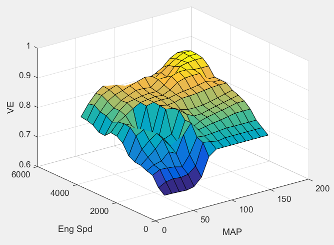#### Dependencies

To enable this parameter, for the Air mass flow model parameter, select ```Simple Speed-Density```.

Intake manifold pressure breakpoints for speed-density volumetric efficiency lookup table, in KPa.

#### Dependencies

To enable this parameter, for the Air mass flow model parameter, select ```Simple Speed-Density```.

Engine speed breakpoints for speed-density volumetric efficiency lookup table, in rpm.

#### Dependencies

To enable this parameter, for the Air mass flow model parameter, select ```Simple Speed-Density```.

The cylinder volume at intake valve close table (IVC), ${f}_{Vivc}$ is a function of the intake cam phaser angle

`${V}_{IVC}={f}_{Vivc}\left({\phi }_{ICP}\right)$`

where:

• ${V}_{IVC}$ is cylinder volume at IVC, in L.

• ${\phi }_{ICP}$ is intake cam phaser angle, in crank advance degrees.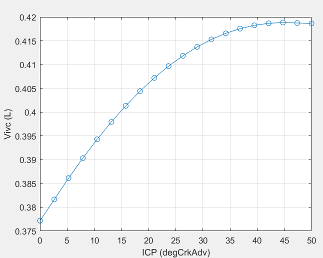#### Dependencies

To enable this parameter, for the Air mass flow model parameter, select ```Dual-Independent Variable Cam Phasing```.

Cylinder volume intake cam phase breakpoints, in L.

#### Dependencies

To enable this parameter, for the Air mass flow model parameter, select ```Dual-Independent Variable Cam Phasing```.

The trapped mass correction factor table, ${f}_{TMcorr}$, is a function of the normalized density and engine speed

where:

• $T{M}_{corr}$, is trapped mass correction multiplier, dimensionless.

• ${\rho }_{norm}$ is normalized density, dimensionless.

• N is engine speed, in rpm.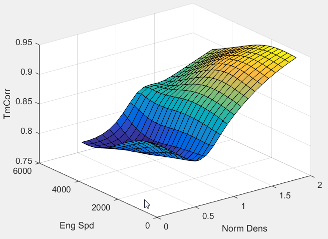#### Dependencies

To enable this parameter, for the Air mass flow model parameter, select ```Dual-Independent Variable Cam Phasing```.

Normalized density breakpoints, dimensionless.

#### Dependencies

To enable this parameter, for the Air mass flow model parameter, select ```Dual-Independent Variable Cam Phasing```.

Engine speed breakpoints, in rpm.

#### Dependencies

To enable this parameter, for the Air mass flow model parameter, select ```Dual-Independent Variable Cam Phasing```.

The phaser intake mass flow model lookup table is a function of exhaust cam phaser angles and trapped air mass flow

`${\stackrel{˙}{m}}_{intkideal}={f}_{intkideal}\left({\phi }_{ECP},T{M}_{flow}\right)$`

where:

• ${\stackrel{˙}{m}}_{intkideal}$ is engine intake port mass flow at arbitrary cam phaser angles, in g/s.

• ${\phi }_{ECP}$ is exhaust cam phaser angle, in degrees crank retard.

• $T{M}_{flow}$ is flow rate equivalent to corrected trapped mass at the current engine speed, in g/s.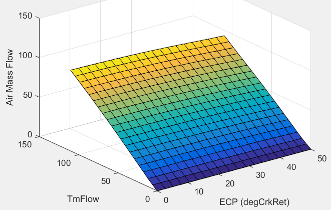#### Dependencies

To enable this parameter, for the Air mass flow model parameter, select ```Dual-Independent Variable Cam Phasing```.

Exhaust cam phaser breakpoints for air mass flow lookup table, in degrees crank retard.

#### Dependencies

To enable this parameter, for the Air mass flow model parameter, select ```Dual-Independent Variable Cam Phasing```.

Trapped mass flow breakpoints for air mass flow lookup table, in g/s.

#### Dependencies

To enable this parameter, for the Air mass flow model parameter, select ```Dual-Independent Variable Cam Phasing```.

The intake air mass flow correction lookup table, ${f}_{aircorr}$, is a function of ideal load and engine speed

`${\stackrel{˙}{m}}_{air}={\stackrel{˙}{m}}_{intkideal}{f}_{aircorr}\left({L}_{ideal},N\right)$`

where:

• ${L}_{ideal}$ is engine load (normalized cylinder air mass) at arbitrary cam phaser angles, uncorrected for final steady-state cam phaser angles, dimensionless.

• N is engine speed, in rpm.

• ${\stackrel{˙}{m}}_{air}$ is engine intake air mass flow final correction at steady-state cam phaser angles, in g/s.

• ${\stackrel{˙}{m}}_{intkideal}$ is engine intake port mass flow at arbitrary cam phaser angles, in g/s.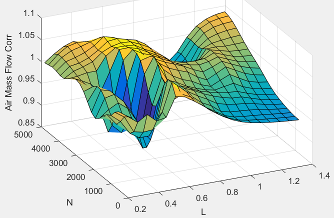#### Dependencies

To enable this parameter, for the Air mass flow model parameter, select ```Dual-Independent Variable Cam Phasing```.

Engine load breakpoints for air mass flow final correction, dimensionless.

#### Dependencies

To enable this parameter, for the Air mass flow model parameter, select ```Dual-Independent Variable Cam Phasing```.

Engine speed breakpoints for air mass flow final correction, in rpm.

#### Dependencies

To enable this parameter, for the Air mass flow model parameter, select ```Dual-Independent Variable Cam Phasing```.

Torque

For the simple torque lookup table model, the SI engine uses a lookup table map that is a function of engine speed and load, ${T}_{brake}={f}_{TnL}\left(L,N\right)$, where:

• ${T}_{brake}$ is engine brake torque after accounting for spark advance, AFR, and friction effects, in N·m.

• L is engine load, as a normalized cylinder air mass, dimensionless.

• N is engine speed, in rpm.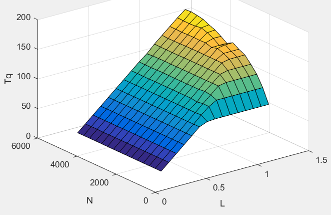The simple torque lookup model assumes that the calibration has negative torque values to indicate the non-firing engine load (L) versus speed (N) condition. The calibrated table (L-by-N) contains the non-firing data in the first table row (1-by-N). When the fuel delivered to the engine is zero, the model uses the data in the first table row (1-by-N) at or above 100 AFR. 100 AFR results from fuel cutoff or very lean operation where combustion cannot occur.

#### Dependencies

To enable this parameter, for the Torque model parameter, select `Simple Torque Lookup`.

#### Dependencies

To enable this parameter, for the Torque model parameter, select `Simple Torque Lookup`.

Engine speed breakpoints, N, in rpm.

#### Dependencies

To enable this parameter, for the Torque model parameter, select `Simple Torque Lookup`.

If you select Crank angle pressure and torque on the block Torque tab, you can:

• Simulate advanced closed-loop engine controls in desktop simulations and on HIL bench, based on cylinder pressure recorded from a model or laboratory test as a function of crank angle.

• Simulate driveline vibrations downstream of the engine due to high-frequency crankshaft torsionals.

• Simulate engine misfires due to lean operation or spark plug fouling by using the injector pulse width input.

• Simulate cylinder deactivation effect (closed intake and exhaust valves, no injected fuel) on individual cylinder pressures, mean-value airflow, mean-value torque, and crank-angle-based torque.

• Simulate the fuel-cut effect on individual cylinder pressure, mean-value torque, and crank-angle-based torque.

#### Dependencies

To enable this parameter, set Torque model to `Torque Structure`.

Cylinder pressure table Prs, as a function of speed N, load L, and crank angle M, in Pa.

#### Dependencies

To enable this parameter, for the Torque model parameter, select `Torque Structure`. Select Crank angle pressure and torque.

Brake torque table Tbrake, as a function of speed N, load L, and crank angle M, in N·m.

#### Dependencies

To enable this parameter, for the Torque model parameter, select `Torque Structure`. Select Crank angle pressure and torque.

Speed breakpoints, N, in rpm.

#### Dependencies

To enable this parameter, for the Torque model parameter, select `Torque Structure`. Select Crank angle pressure and torque.

#### Dependencies

To enable this parameter, for the Torque model parameter, select `Torque Structure`. Select Crank angle pressure and torque.

Crank angle breakpoints, M, in deg.

#### Dependencies

To enable this parameter, for the Torque model parameter, select `Torque Structure`. Select Crank angle pressure and torque.

Top dead center (TDC) compression angles by cylinder, in deg.

#### Dependencies

To enable this parameter, for the Torque model parameter, select `Torque Structure`. Select Crank angle pressure and torque.

The inner torque lookup table, ${f}_{Tqinr}$, is a function of engine speed and engine load, $T{q}_{inr}={f}_{Tqinr}\left(L,N\right)$, where:

• $T{q}_{inr}$ is inner torque based on gross indicated mean effective pressure, in N·m.

• L is engine load at arbitrary cam phaser angles, corrected for final steady-state cam phaser angles, dimensionless.

• N is engine speed, in rpm.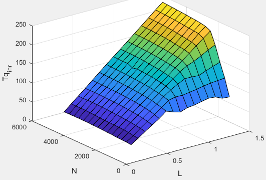#### Dependencies

To enable this parameter, for the Torque model parameter, select `Torque Structure`.

The friction torque lookup table, ${f}_{Tfric}$, is a function of engine speed and engine load, ${T}_{fric}={f}_{Tfric}\left(L,N\right)$, where:

• ${T}_{fric}$ is friction torque offset to inner torque, in N·m.

• L is engine load at arbitrary cam phaser angles, corrected for final steady-state cam phaser angles, dimensionless.

• N is engine speed, in rpm.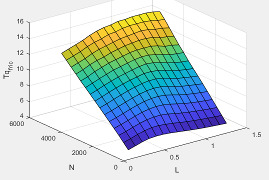#### Dependencies

To enable this parameter, for the Torque model parameter, select `Torque Structure`.

Engine temperature modifier on friction torque, ƒfric,temp, dimensionless.

#### Dependencies

To enable this parameter, for the Torque model parameter, select `Torque Structure`.

Engine temperature modifier breakpoints, in K.

#### Dependencies

To enable this parameter, for the Torque model parameter, select `Torque Structure`.

The pumping work lookup table, ƒTpump, is a function of engine load and engine speed, `Tpump=ƒTpump(L,N)`, where:

• Tpump is pumping work, in N·m.

• L is engine load, as a normalized cylinder air mass, dimensionless.

• N is engine speed, in rpm.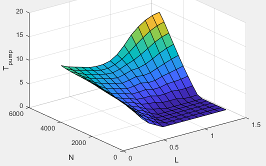#### Dependencies

To enable this parameter, for the Torque model parameter, select `Torque Structure`.

The optimal spark lookup table, ${f}_{SAopt}$, is a function of engine speed and engine load, $S{A}_{opt}={f}_{SAopt}\left(L,N\right)$, where:

• SAopt is optimal spark advance timing for maximum inner torque at stoichiometric air-fuel ratio (AFR), in deg.

• L is engine load at arbitrary cam phaser angles, corrected for final steady-state cam phaser angles, dimensionless.

• N is engine speed, in rpm.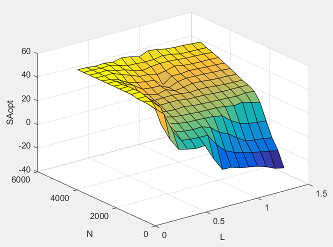#### Dependencies

To enable this parameter, for the Torque model parameter, select `Torque Structure`.

#### Dependencies

To enable this parameter, for the Torque model parameter, select `Torque Structure`.

Inner torque speed breakpoints, in rpm.

#### Dependencies

To enable this parameter, for the Torque model parameter, select `Torque Structure`.

The spark efficiency lookup table, ${f}_{Msa}$, is a function of the spark retard from optimal

`$\begin{array}{l}{M}_{sa}={f}_{Msa}\left(\Delta SA\right)\\ \Delta SA=S{A}_{opt}-SA\end{array}$`

where:

• ${M}_{sa}$ is the spark retard efficiency multiplier, dimensionless.

• $\Delta SA$is the spark retard timing distance from optimal spark advance, in deg.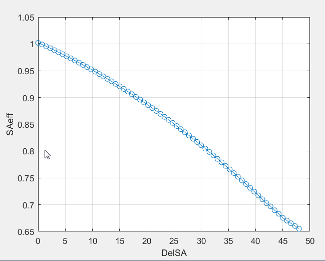#### Dependencies

To enable this parameter, for the Torque model parameter, select `Torque Structure`.

Spark retard from optimal inner torque timing breakpoints, in deg.

#### Dependencies

To enable this parameter, for the Torque model parameter, select `Torque Structure`.

The lambda efficiency lookup table, ${f}_{M\lambda }$, is a function of lambda, ${M}_{\lambda }={f}_{M\lambda }\left(\lambda \right)$, where:

• ${M}_{\lambda }$ is the lambda multiplier on inner torque to account for the air-fuel ratio (AFR) effect, dimensionless.

• $\lambda$ is lambda, AFR normalized to stoichiometric fuel AFR, dimensionless.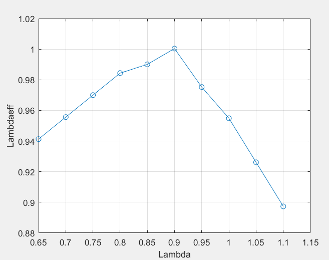#### Dependencies

To enable this parameter, for the Torque model parameter, select `Torque Structure`.

Lambda effect on inner torque lambda breakpoints, dimensionless.

#### Dependencies

To enable this parameter, for the Torque model parameter, select `Torque Structure`.

Exhaust

The exhaust temperature lookup table, ${f}_{Texh}$, is a function of engine load and engine speed

`${T}_{exh}={f}_{Texh}\left(L,N\right)$`

where:

• Texh is engine exhaust temperature, in K.

• L is normalized cylinder air mass or engine load, dimensionless.

• N is engine speed, in rpm.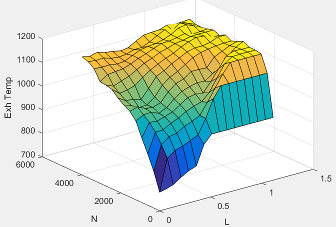Engine load breakpoints used for exhaust temperature lookup table, dimensionless.

Engine speed breakpoints used for exhaust temperature lookup table, in rpm.

Exhaust gas-specific heat, $C{p}_{exh}$, in J/(kg·K).

The SI Core Engine CO2 emission mass fraction lookup table is a function of engine torque and engine speed, CO2 Mass Fraction = ƒ(Speed, Torque), where:

• CO2 Mass Fraction is the CO2 emission mass fraction, dimensionless.

• Speed is engine speed, in rpm.

• Torque is engine torque, in N·m.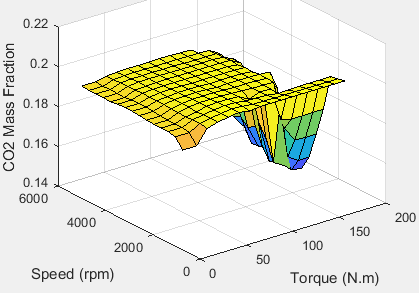#### Dependencies

To enable this parameter, on the Exhaust tab, select CO2.

The SI Core Engine CO emission mass fraction lookup table is a function of engine torque and engine speed, CO Mass Fraction = ƒ(Speed, Torque), where:

• CO Mass Fraction is the CO emission mass fraction, dimensionless.

• Speed is engine speed, in rpm.

• Torque is engine torque, in N·m.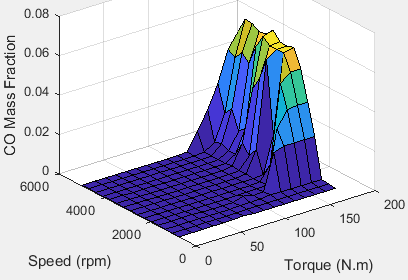#### Dependencies

To enable this parameter, on the Exhaust tab, select CO.

The SI Core Engine HC emission mass fraction lookup table is a function of engine torque and engine speed, HC Mass Fraction = ƒ(Speed, Torque), where:

• HC Mass Fraction is the HC emission mass fraction, dimensionless.

• Speed is engine speed, in rpm.

• Torque is engine torque, in N·m.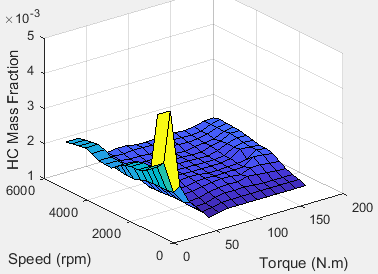#### Dependencies

To enable this parameter, on the Exhaust tab, select HC.

The SI Core Engine NOx emission mass fraction lookup table is a function of engine torque and engine speed, NOx Mass Fraction = ƒ(Speed, Torque), where:

• NOx Mass Fraction is the NOx emission mass fraction, dimensionless.

• Speed is engine speed, in rpm.

• Torque is engine torque, in N·m.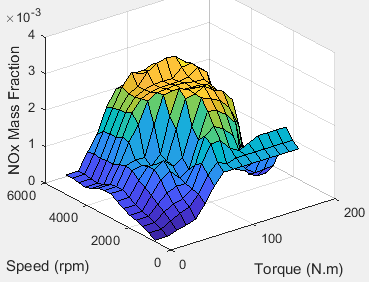#### Dependencies

To enable this parameter, on the Exhaust tab, select NOx.

The SI Core Engine PM emission mass fraction lookup table is a function of engine torque and engine speed where:

• PM is the PM emission mass fraction, dimensionless.

• Speed is engine speed, in rpm.

• Torque is engine torque, in N·m.

#### Dependencies

To enable this parameter, on the Exhaust tab, select PM.

Engine speed breakpoints used for the emission mass fractions lookup tables, in rpm.

#### Dependencies

To enable this parameter, on the Exhaust tab, select CO2, CO, NOx, HC, or PM.

Engine torque breakpoints used for the emission mass fractions lookup tables, in N·m.

#### Dependencies

To enable this parameter, on the Exhaust tab, select CO2, CO, NOx, HC, or PM.

Fuel

Fuel injector slope, ${S}_{inj}$, mg/ms.

Air-fuel ratio, $AFR$.

Fuel lower heating value, LHV, in J/kg.

Specific gravity of fuel, Sgfuel, dimensionless.

 Gerhardt, J., Hönninger, H., and Bischof, H., A New Approach to Functional and Software Structure for Engine Management Systems — BOSCH ME7. SAE Technical Paper 980801, 1998.

 Heywood, John B. Internal Combustion Engine Fundamentals. New York: McGraw-Hill, 1988.

## SupportGet trial now